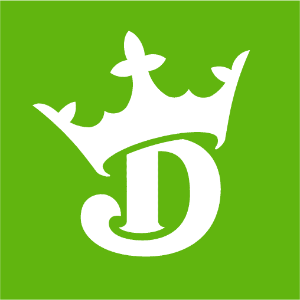DraftKings 2019 Stanley Cup Odds
+800
8 to 1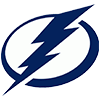Lightning
8.6% implied probability

+950
9.5 to 1Bruins
7.4% implied probability

+1000
10 to 1Avalanche
7.0% implied probability

+1000
10 to 1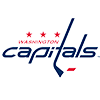Capitals
7.0% implied probability

+1000
10 to 1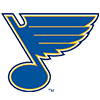Blues
7.0% implied probability

+1300
13 to 1Penguins
5.5% implied probability

+1300
13 to 1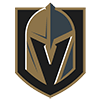Golden Knights
5.5% implied probability

+1300
13 to 1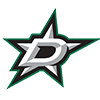Stars
5.5% implied probability

+1400
14 to 1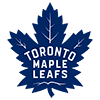Maple Leafs
5.2% implied probability

+1600
16 to 1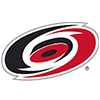Hurricanes
4.6% implied probability

+1800
18 to 1Coyotes
4.1% implied probability

+1800
18 to 1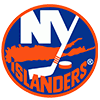Islanders
4.1% implied probability

+2400
24 to 1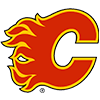Flames
3.1% implied probability

+2500
25 to 1Predators
3.0% implied probability

+3000
30 to 1Canucks
2.5% implied probability

+3000
30 to 1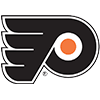Flyers
2.5% implied probability

+3000
30 to 1Oilers
2.5% implied probability

+3000
30 to 1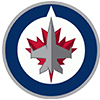Jets
2.5% implied probability

+3700
37 to 1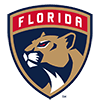Panthers
2.0% implied probability

+4000
40 to 1Wild
1.9% implied probability

+5500
55 to 1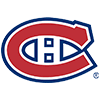Canadiens
1.4% implied probability

+6000
60 to 1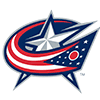Blue Jackets
1.3% implied probability

+6500
65 to 1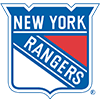Rangers
1.2% implied probability

+7000
70 to 1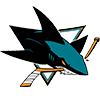Sharks
1.1% implied probability

+8000
80 to 1Sabres
1.0% implied probability

+9000
90 to 1Ducks
0.9% implied probability

+10000
100 to 1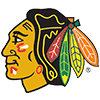Blackhawks
0.8% implied probability

+22500
225 to 1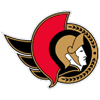Senators
0.3% implied probability

+30000
300 to 1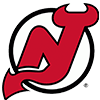Devils
0.3% implied probability

+30000
300 to 1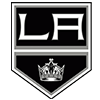Kings
0.3% implied probability

+150000
1500 to 1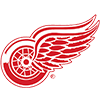Red Wings
0.1% implied probability